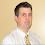Thoughts, Tips and Tricks on what I'm currently do for a living. Currently most of my spare time is spent on contributing to Akka.NET.

## Sunday, October 26, 2014

### Integration Testing using Akka.NET's TestKit

In Getting Started with Akka.NET and Handling messages and state with Akka.NET we created a calculator in Akka.NET that was able to add and subtract numbers and return the answer. It also stores the last answer and can respond with it when asked.

In Unit Testing using Akka.NET’s TestKit we created some unit tests to verify internal state of the actor.

In this post we’ll switch to integration tests – verifying we get correct messages back.

All posts in this series: Tutorials for Akka.NET

Note! This post was written using Akka.NET 0.7.0 and might not work for later versions

### Unit Testing Recap

Unit Testing using Akka.NET’s TestKit we created some unit tests to verify internal state of the actor. We used `ActorOfAsTestActorRef<T>()` which creates the actor in the test’s `ActorSystem` (which is exposed thru the property `Sys` inside tests) and returns a `TestActorRef<T>` which give us access to the underlycing actor instance using the property `UnderlyingActor`.

``````[Fact]
{
TestActorRef<CalculatorActor> calculatorRef = ActorOfAsTestActorRef<CalculatorActor>("calculator");
CalculatorActor calculator = calculatorRef.UnderlyingActor;
}

[Fact]
{
TestActorRef<CalculatorActor> calculatorRef = ActorOfAsTestActorRef<CalculatorActor>("calculator");
CalculatorActor calculator = calculatorRef.UnderlyingActor;
}``````

Remember that everything is synchronous when writing these kind of tests. It’s the use of `ActorOfAsTestActorRef<T>()` that makes it synchronous. A `CallingThreadDispatcher` is used for actors created using `ActorOfAsTestActorRef<T>()` so when we send it a message using `Tell(message)` it’s not dispatched on another thread, but instead immediately processed before `Tell` returns control back to our test.

### Integration tests

When writing integration tests, we create and run the actor the way we normally do. This means multi threaded under full concurrency (actors are shielded by the Actor model – the actor only processes on message at a time).

In our first unit test we verfied that the internal state `answer` is 0 initially. We can also verify this by sending the actor a `GetLastAnswer` message and verify that we get an `Answer(0)` back.

The skeleton for the test looks like this:

``````public class CalculatorIntegrationTests : TestKit
{
[Fact]
{
var calculator = ActorOf<CalculatorActor>("calculator");

//Somehow verify we get an Anser(0) back
}
}``````

So how can we verify that the calculator responds with what we expect? We could use `Ask` but there is a better way using TestKit.

### TestActor

When the TestKit’s `ActorSystem` is created it also creates a special actor called `TestActor`. This instance is used as an implicit sender, so when we sent a message to calculator like this:

``calculator.Tell(GetLastAnswer.Instance);``

It was actually sent as if we’d specified `TestActor` as the sender:

``calculator.Tell(GetLastAnswer.Instance, TestActor);``

So when calculator send the response it will send it to `TestActor` which in turn will put the message in a queue that we can test against.

To test that the queue contains the correct message, or another way of seeing it: that `TestActor` received the correct message we use `ExpectMessage<T>()` and then assert that the value is correct.

``````[Fact]
{
var calculator = ActorOf<CalculatorActor>("calculator");

}``````

The last two lines can also be written like this:

``ExpectMsg<Answer>(a => a.Value == 0);``

### Isn’t it asynchronous?

We send a message to `calculator` which will process the message on another thread. At the same time we test that we have received a response. How can we be sure that the calculator has responded before we execute `ExpectMessage<Answer>()`? Don’t we need to synchronize somehow? Or is ir running synchronously as with unit tests?

No, it really is asynchronous, BUT `ExpectMsg` will wait up to 3 seconds before it fails.

Rewriting the second unit test as a integration test is really easy now that we have all building blocks.

``````    [Fact]
{
var calculator = ActorOf<CalculatorActor>("calculator");

}``````

Be aware that the tests run in a full `ActorSystem` and everything is asynchronous even though it might look like synchronous code.

### Code

All posts in this series: Tutorials for Akka.NET

#### 1 comment:

1.After reading your posts I was reminder of a Gregg Young video that I think ties into Actors and testing.

https://skillsmatter.com/skillscasts/1947-talk-from-greg-young